# Negative Numbers Worksheet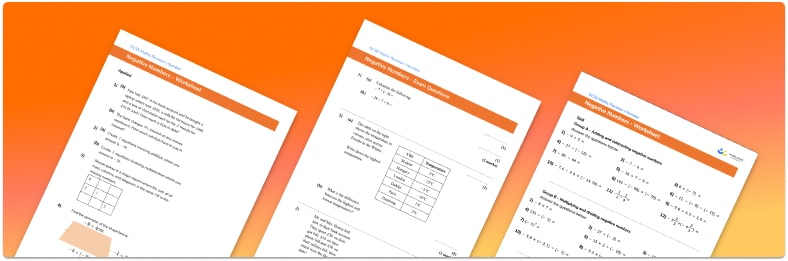• Section 1 of the negative numbers worksheet contains 36 skills based negative numbers questions, in 3 groups to support differentiation
• Section 2 contains 4 applied negative numbers questions with a mix of worded problems and deeper problem solving questions
• Section 3 contains 4 foundation and higher level GCSE exam style negative numbers questions
• Answers and a mark scheme for all negative numbers questions are provided
• Questions follow variation theory with plenty of opportunities for students to work independently at their own level
• All questions are created by fully qualified expert secondary maths teachers
• Suitable for GCSE maths revision for AQA, OCR and Edexcel exam boards

• This field is for validation purposes and should be left unchanged.

You can unsubscribe at any time (each email we send will contain an easy way to unsubscribe). To find out more about how we use your data, see our privacy policy.

### Negative numbers a glance

Negative numbers are numbers that are below zero such as -1, -2, … etc. Negative numbers are denoted by a minus sign in front of the number.

Negative integers are shown on the left of the zero on a number line and a negative number line can be a useful tool when comparing negative numbers and ordering negative numbers.  Negative number lines can also help us when we are learning to calculate with negative numbers.

We can add, subtract, multiply and divide negative numbers and there are specific rules for this. When adding and subtracting negative numbers, we find the starting point and move up the number line for addition and down for subtraction, in the same way we do when calculating with positive numbers. When multiplying and dividing negative numbers, multiplying two negative numbers gives a positive answer and multiplying a negative number by a positive number gives a negative answer.

Looking forward, students can then progress to additional number worksheets, for example aor aFor more teaching and learning support on Number our GCSE maths lessons provide step by step support for all GCSE maths concepts.

## Related worksheets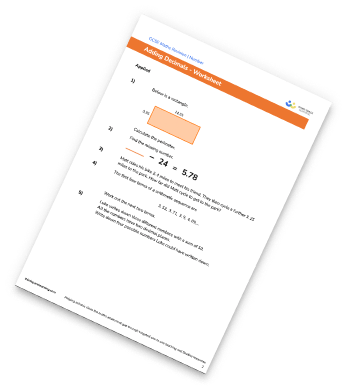Subtracting decimals worksheet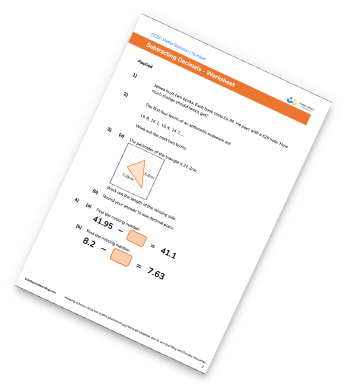Ordering fractions worksheet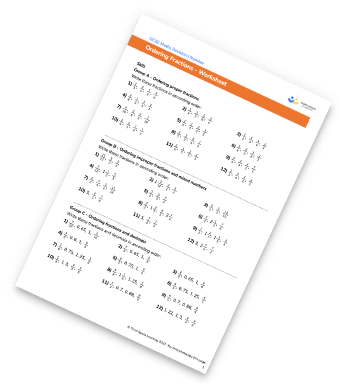Converting Mixed Numbers To Improper Fractions Worksheet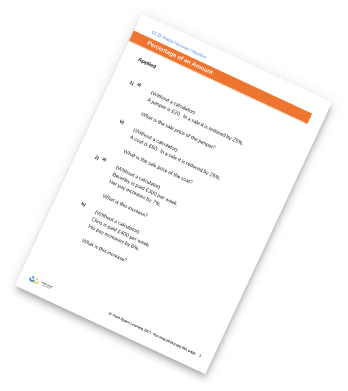## Do you have KS4 students who need more focused attention to succeed at GCSE?There will be students in your class who require individual attention to help them succeed in their maths GCSEs. In a class of 30, it’s not always easy to provide.

Help your students feel confident with exam-style questions and the strategies they’ll need to answer them correctly with our dedicated GCSE maths revision programme.

Lessons are selected to provide support where each student needs it most, and specially-trained GCSE maths tutors adapt the pitch and pace of each lesson. This ensures a personalised revision programme that raises grades and boosts confidence.

Find out more